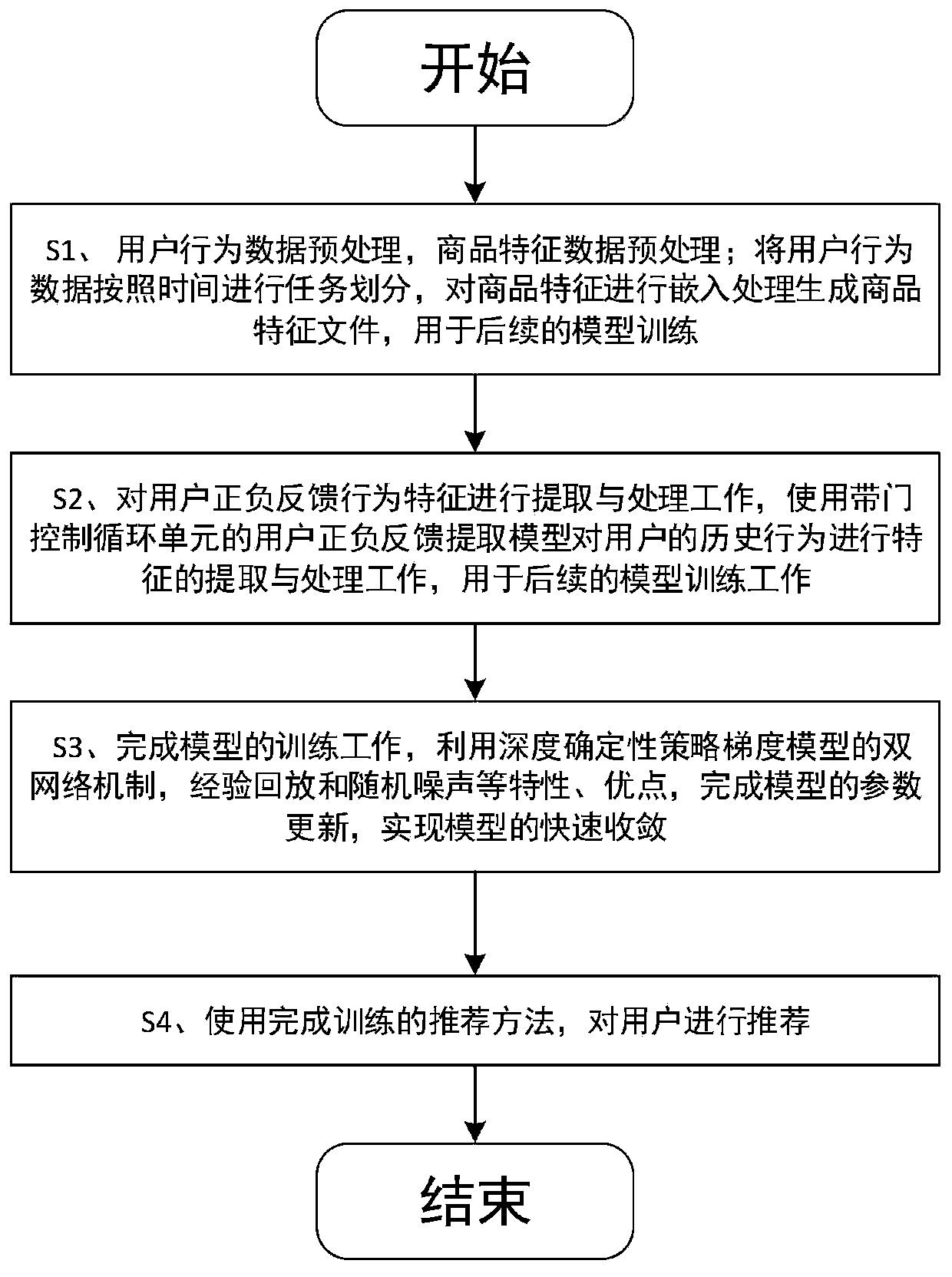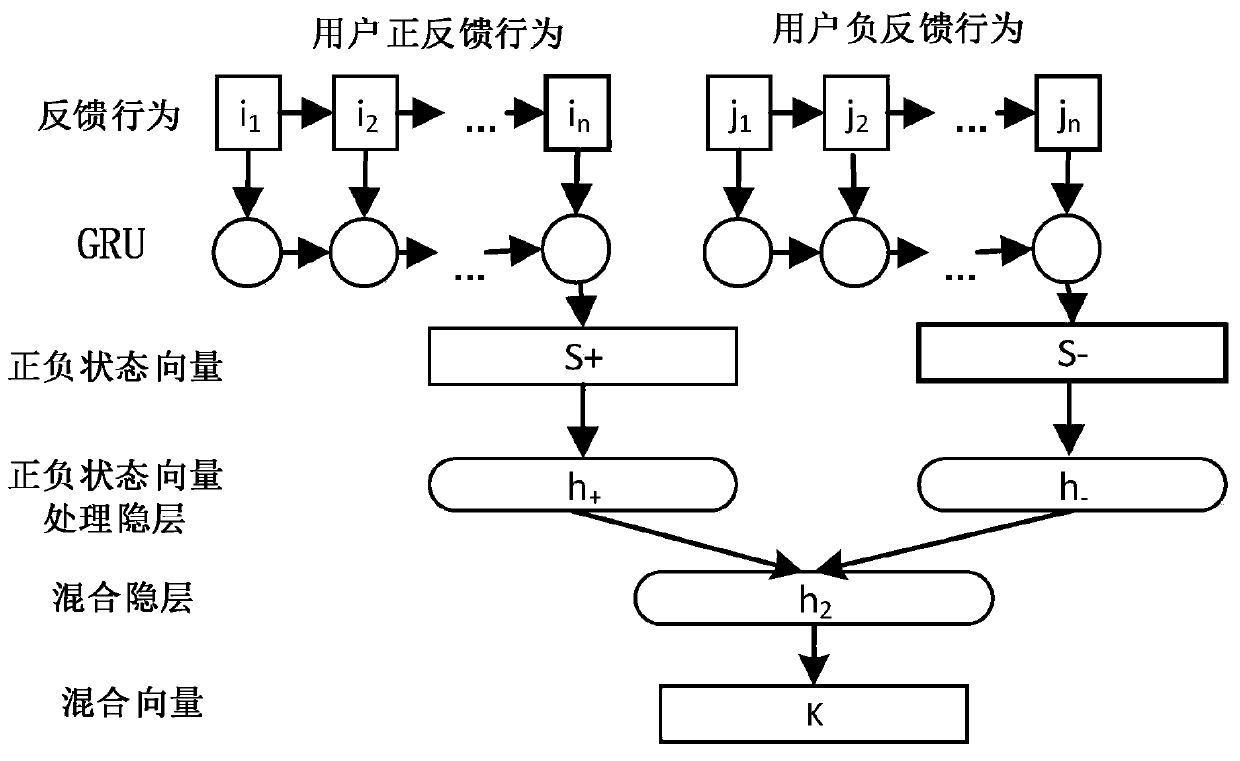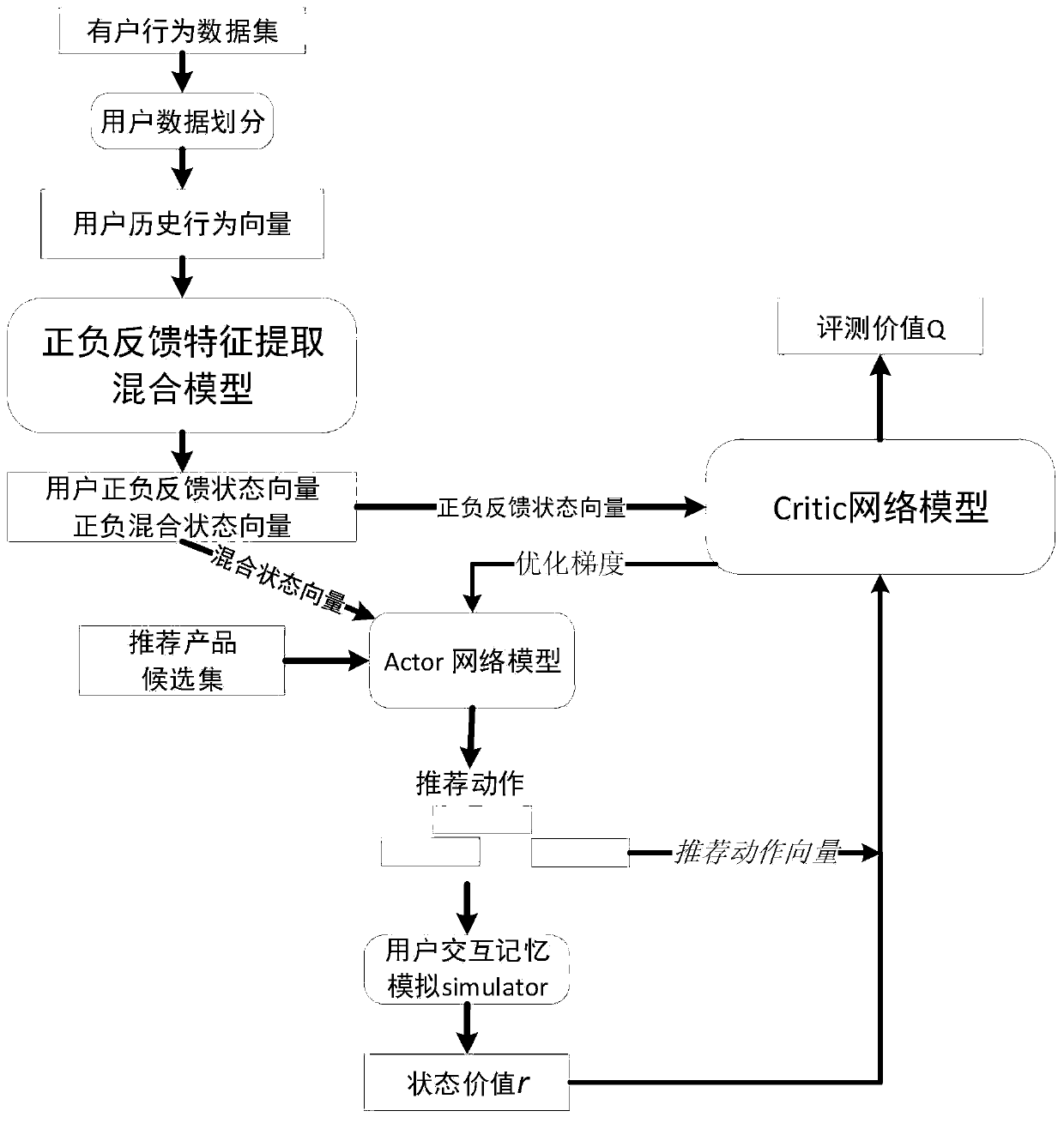# Deep reinforcement learning-based recommendation method and system with negative feedback

## A technology of reinforcement learning and recommendation methods, applied in neural learning methods, data processing applications, biological neural network models, etc., can solve the problems of slow learning rate and low accuracy

Pending Publication Date: 2020-08-11
HUAZHONG UNIV OF SCI & TECH
11 Cites 3 Cited by

## AI-Extracted Technical Summary

### Problems solved by technology

 In view of the above defects or improvement needs of the prior art, the present invention provides a recommendation method and system based on deep re...
View more

## Abstract

The invention discloses a deep reinforcement learning-based recommendation method and system with negative feedback, and the method comprises the steps: collecting commodity feature information, and collecting user behavior data to obtain positive and negative feedback behavior vectors of a user; performing feature extraction on the positive and negative feedback behavior vectors of the user through a feature extraction network model to obtain a positive and negative feedback feature mixed state vector of the user; training a deep deterministic strategy gradient model composed of a strategy network and an estimation network by using the positive and negative feedback feature mixed state vector of the user until the model converges; and generating a positive and negative feedback feature mixed state vector according to historical behaviors of a user needing work recommendation, and generating a user recommendation commodity list for the user for selection through the trained deep deterministic strategy gradient model to complete the user work recommendation. The parameter updating of the related neural network can be delayed, so that the correlation between the networks is reduced,and the training speed and accuracy of the recommendation method are improved.

Application Domain

Neural architecturesNeural learning methods +1

Technology Topic

Network modelEngineering +6

## Image

•••## Examples

• Experimental program(1)

### Example Embodiment

 In order to make the objectives, technical solutions and advantages of the present invention clearer, the following further describes the present invention in detail with reference to the accompanying drawings and embodiments. It should be understood that the specific embodiments described herein are only used to explain the present invention, but not to limit the present invention. In addition, the technical features involved in the various embodiments of the present invention described below can be combined with each other as long as they do not conflict with each other.
 The present invention provides a method such as figure 1 As shown, specifically including:
 S1. The data acquisition based on the deep reinforcement learning recommendation method with negative feedback mainly depends on the behavior information of the user in the e-commerce website when visiting the website and the characteristic information of the product. After obtaining the data on the real e-commerce website, the product characteristic information needs to be measured. Extract and divide user behavior data;
 S1.1. According to the collected product feature information, it is divided into time and product category, and all features of the product are counted to filter out the appropriate number of product features and have a high coverage of all product categories and meaningful product features to generate a feature dictionary. Then, different commodities at different time points are embedding according to the feature dictionary to obtain the commodity feature vector at each moment, and generate the commodity feature vector set embed file. After the processing is completed, the data format is (timestamp, itemid, embedding). Among them, embedding is the feature vector of the product, which is used for subsequent training and recommendation work.
 S1.2. According to the collected user behavior data, the user behavior data set is obtained by dividing according to the behavior time, user name, behavior action, and product number, and the format of the processing completed is (timestamp, visitorid, event, itemid). Divide the processed behavior data set according to users and time to obtain the user behavior vector U t ={{i 1 , I 2 ,..., i n }, {j 1 , J 2 ,..., j n }}, where in and jn are the product numbers for which the user has received positive feedback and negative feedback in a certain period of time.
 S2. After processing the user's positive and negative feedback behavior vector through the feature extraction network model, the user's positive and negative feedback feature mixed state vector is obtained. The feature extraction network model structure is as follows figure 2 As shown; the preliminary preparations include the completion of the Gated Recurrent Units (Gated Recurrent Units, GRU) user positive and negative feedback state vector extraction network model parameter initialization, user simulation memory simulator initialization, memory pool replay buffer initialization and other tasks, and then perform user positive The generation of negative feedback state vector:
 S2.1. In the recommendation method with negative feedback based on deep reinforcement learning, according to the user historical behavior vector U generated in step S1.2 t ={{i 1 , I 2 ,..., i n }, {j 1 , J 2 ,..., j n }} Generate user positive feedback state vector S t+ ={s 1+ , S 2+ ,...,S n+ } And the negative feedback state vector S t- ={s 1- , S 2- ,...,S n- }, choose to use the recurrent neural network with GRU to complete the processing work. The reason for choosing the GRU unit is because compared to the Long Short-Term Memory (LSTM), the GRU unit is shaping the user's continuous behavior state S t There will be advantages.
 In the behavior vector generation RNN network, the GRU unit will use the update gate z n To generate a new state and use the reset gate r n To control the output h from the previous GRU unit in the RNN network n-1 , The user’s positive behavior {i 1 , I 2....i n } (Negative behavior processing process is the same) input into RNN, the processing process formula (1-1)-(1-4) shows:
 z n =σ(W z i n +U z h n-1 ), (1-1)
 r n =σ(W r i n +U r h n-1 ), (1-2)
 h′ n =tanh[Wi n +U(r n ·H n-1 )], (1-3)
 h n =(1-z n )h n-1 +z n h′ n , (1-4)
 σ(), tanh() is the nonlinear activation function, W z , W r , W is the weight value of the corresponding layer, U z , U r , U is the linear layer.
 S2.2. Input the positive and negative feedback state vector into the corresponding processing hidden layer, and then output the latter two processing hidden layers to the fully connected hybrid hidden layer to generate the positive and negative feedback mixed state vector K t ={k 1 , K 2 ,..., k n }, the process is shown in formulas (1-5)-(1-7):
 h 1 =w 1 S t+ +b 1 (1-5)
 h 2 =w 2 S t- +b 2 (1-6)
 K t =W + h 1 +W - h 2 +b (1-7)
 Where W 1 , W 2 , W + , W - Are the weight matrices corresponding to the positive and negative feedback vectors, b 1 , B 2 , B is the bias matrix, t is a certain moment.
 S3. Complete the training of the recommended method, the specific process is as follows image 3 As shown; the model parameters of the training model need to be initialized in advance. The parameters include dual Actor strategy network (online Actor, target Actor) and dual Critic value network (online Critic, target Critic) parameters, the number of iterations of the network model, and the recommended behavior vector length , Batch_size, and the learning rate of the model, and then complete the model training.
 S3.1. The mixed state vector K t ={k 1 , K 2 ,..., k n } Input to the online Actor network to generate recommended action A t ={a 1 , A 2 ,..., a k }, and the user’s recommendation action A t Feedback, using user interaction memory simulator generated in K t State A t After the state value r t , Generate a new user behavior vector U t+1 , Save the result to the memory pool, complete the memory pool data update:
 Use steps S2.1-S2.2 to generate the mixed state vector K t ={k 1 , K 2 ,..., k n } And input it into the online Actor network, the online Actor network generates recommended actions according to Algorithm 1.1; the specific process is, according to the strategy function And the current mixed state vector K t ={k 1 , K 2 ,..., k n }, generate weight vector W t ={w 1 , W 2 ,..., w k }, where the strategy function Is about the parameter θ π Function, its function is to mix the feature vector K t Mapped into the weight space, the present invention selects the Actor strategy deep neural network to implement the strategy function here The function, the process is shown in formula (1-8):

 According to the generated W t ={w 1 , W 2 ,..., w k }中w i And the product feature vector E in the recommended product candidate set I i ={e 1 , E 2 ,..., e n } Do the dot multiplication to generate the score socre E i , The process is shown in formula (1-9):
 socre E i =w k t E T i (1-9)
 Will score socre E i The highest product Ei is added to A t In, generate recommended action vector A t ={a 1 , A 2 ,..., a k }, the specific algorithm is shown in Table 1:
 Table 1


 And calculate the current behavior vector U according to the user simulation memory simulator t , Recommended action A t , Calculate the similarity with the historical behavior in the memory pool Cosine(p t , M i ), as shown in formula (1-10):

 Where α is the conversion rate of the behavior state, p t Is the similarity with mi records in the memory pool, u i With a i M in the memory pool i The historical behavior vector and the recommended behavior vector in the record.
 Then use the Cosine(p t , M i ) Is normalized, as shown in formula (1-11):

 Where M is all the records in the memory pool, m j Is the record in M, r i Is the i-th value.
 Get the state value r in the current state t Value, as shown in formula (1-12):
 r t =∑m j ∈M P(p t →r i )*r i (1-12)
 According to the state value r t Value will be the current recommended action A t Add to {i 1 , I 2 ,..., i n } And {j 1 , J 2 ,..., j n }, if r t 0 then A t Add to the user's positive behavior to generate user behavior U t+1 ={{i 1 , I 2 ,..., i n , A t }, {j 1 , J 2 ,..., j n }}, otherwise it will be added to the user’s negative behavior to generate U t+1 ={{i 1 , I 2 ,..., i n }, {j 1 , J 2 ,..., j n , A t }} and put (U t , A t , R t , U t+1 ) The record is added to the memory pool for subsequent model training and learning.
 S3.2. Randomly select batch size records from the memory pool for model training, and use steps S2.1, S2.2 to generate positive and negative feedback state vectors S t ={S t+ , S t- }, S t+1 ={S t+1+ , S t+1- } And the mixed state vector K t With K t+1 , State value r t Complete model training;
 S3.3. Use the positive and negative feedback mixed state vector K in step S3.2 t+1 , Target Actor network uses strategy function according to algorithm 1.1 According to K t+1 Generate A t+1;
 S3.4. Use the positive and negative feedback mixed state vector K in step S3.2 t , The online Actor network uses the strategy function according to Algorithm 1.1 According to K t Generate A t;
 S3.5. Change A in steps S3.3 and S3.2 t+1 , S t+1 ={S t+1+ , S t+1- }Delivered to the target Critic value network, the target Critic value network needs to feed back the positive and negative state vector S t+1 ={S t+1+ , S t+1- } And recommended action vector A t+1 ={a 1 , A 2 ,..., a k } Perform corresponding processing, first use the fusion hidden layer to fuse the positive and negative feedback state vectors with the recommended action vector, and then output to the mixed hidden layer for mixing. The process is shown in formulas (1-13)-(1-15) :
 h 1 =w + S t+1+ +w 1a A t+1 +b 1 (1-13)
 h 2 =w - S t+1- +w 2a A t+1 +b 2 (1-14)
 h 3 =w 31 h 1 +w 32 h 2 +b 3 (1-15)
 Where w + , W 1a , W - , W 2a , W 31 , W 32 , B 1 , B 2 , B 3 , Are the weight matrix and the bias matrix respectively.
 target Critic neural network according to h 3 Input to generate the assessed value Q(S t+1 , A t+1;Θ μ’ ) Output, and then Q(S t+1 , A t+1;Θ μ’ ) Is multiplied by the learning rate γ and added based on S t The behavioral value rt of the state, so as to get the actual total value R at time t t , R t The acquisition process is shown in formula (1-16):

 Where E is the expectation.
 S3.6. Change S in steps S3.2 and S3.4 t ={S t+ , S t- }, A t Delivered to the online Critic network, the online Critic network uses the same method as step S3.5 to process S t ={S t+ , S t- }, A t , And then generate predicted behavioral value Q * (S t , A t;Θ μ ), and Q * (S t , A t;Θ μ ) About recommended action A t Gradient direction
 S3.7. Get Q according to steps S3.5 and S3.6 * (S t , A t;Θ μ ) And R t , Calculate the loss function L(θ μ ), the process is shown in formula (1-17):

 Where θ μ It is the online Critic network parameter.
 The online Critic network parameter update is along minimizing(L(θ μ )), the process is shown in formula (1-18):

 S3.8. The target Critic network parameter update form relies on the soft update of the online Critic network parameters and the update rate τ. The process is shown in formula (1-19):
 θ μ’ ←τθ μ +(1-τ)θ μ’ (1-19)
 Where θ μ’ It is the Target Critic network parameter.
 S3.9.Online Actor network parameter update direction is along the strategy function About the online Actor network model parameters and the gradient returned in step S3.6 The optimization gradient direction of is carried out, and the update process is shown in formula (1-20):

 Where θ π It is the online Actor network parameter.
 S3.10. The parameter update form of the target Actor network relies on the soft update of the online Critic network parameters and the update rate τ. The update process is shown in formula (1-21):
 θ π’ ←τθ π +(1-τ)θ π’ (1-21)
 Where θ π’ Is the target Actor network parameter, the specific update process of related network parameters is as Figure 4 Shown.
 S3.11. Combining steps S3.1 to S3.10 is the overall training process of the model, and iterates until the model converges. The specific process is shown in Table 2:
 Table 2



 S4. User product recommendation; according to the user's behavior time and the product area of ​​the e-commerce website that the user is browsing (such as the digital product area, the daily necessities area, the food and drug area, etc.), select all the product features in the product area category at this time period Vector, as a set of commodity feature vectors embed={e 1 , E 2 …, e n }, the embed is delivered to the trained Actor strategy network, and the Actor network generates a state vector S based on the user's historical behavior on the e-commerce website t ={{s 1+ , S 2+ ,...,S n+ }, {s 1- , S 2- ,...,S n- }} and the product feature vector set embed, using algorithm 1.1 to generate a user-recommended product list, for the user to choose (the specific process is the same as step S3.1). Then add the user's positive and negative feedback to the behavior vector to generate u t+1 For subsequent use.
 Those skilled in the art can easily understand that the above descriptions are only preferred embodiments of the present invention and are not intended to limit the present invention. Any modification, equivalent replacement and improvement, etc. made within the spirit and principle of the present invention, All should be included in the protection scope of the present invention.

## PUM## Description & Claims & Application Information

We can also present the details of the Description, Claims and Application information to help users get a comprehensive understanding of the technical details of the patent, such as background art, summary of invention, brief description of drawings, description of embodiments, and other original content. On the other hand, users can also determine the specific scope of protection of the technology through the list of claims; as well as understand the changes in the life cycle of the technology with the presentation of the patent timeline. Login to view more.
Who we serve
• R&D Engineer
• R&D Manager
• IP Professional
Why Eureka